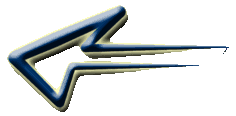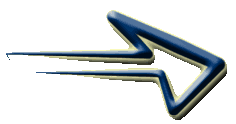# Algebra and Equations

### Algebra is all about solving puzzles with letters, numbers and symbols. It is about finding the unknown by using whatever information you are given.

At first, the whole idea of Algebra can be daunting, which is why a structured approach, taking each step at a time is very important.

I usually get my students to remember three basic rules which I repeat over and over again. With these three rules, some practice and by treating the whole concept as a detective game, most children find Algebra fairly easy.

I'll get to the rules later, the first thing to do is to get your child used to representing numbers with letters and symbols.

These worksheets "Algebra Preparation" and "Algebra Challenges" from
numbers with symbols and they will begin to solve equations
without knowing they are doing it.

Once they are comfortable with this idea, I move on to representing numbers with letters. I start by writing "a = 1, b = 2 and c = 3" and ask questions like -

What is 'a' plus 'b'?  - What is 'b' plus 'c'? - What is 'a' plus 'b' plus 'c'?

Then move on to writing the questions as a calculation with a question mark
a + b = ?,   b + c = ?,   a + b + c ?

When they are happy, I ask "What is 2a?" - this leads to explaining that
writing 2a, really means 2 times 'a' or 2 x 'a' so 2a is 2 x 1 = 2.

Emphasise that a number next to a letter means that the number
and letter are multiplied.

### What is 2a plus 3b? ... 2 x 'a' plus 3 x 'b' ... 2 x 1 plus 3 x 2 .... 2 plus 6 = 8

Be careful to explain that the multiplication must be done before the addition.

I normally experiment with them by writing 2 x 1 + 3 x 2 and work out the plus part first, which gives 2 x 4 x 2 giving the answer 16, which is clearly incorrect.

Ask "How can we make sure that we work out the calculation correctly?" and introduce the idea of making sure that you do any multiplying before adding.

### 2 x 1 plus 3 x 2 ... 2 + 6 = 8

This introduces them to the concept of "Order of Operations" which we will look at later.

This Algebra worksheet from Primary Resources, will allow your child to practice this.

##Balancing Equations______________________________________________________